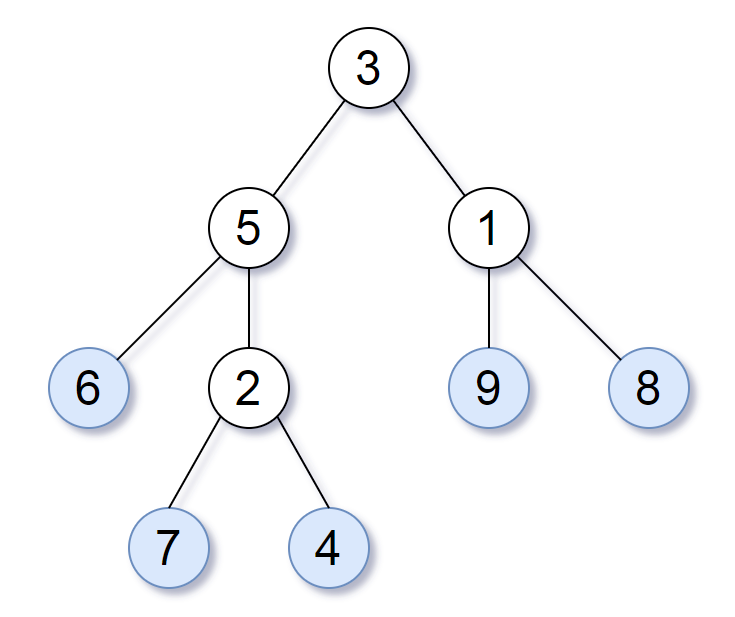LeetCode - Tree - Leaf-Similar Trees

Consider all the leaves of a binary tree.  From left to right order, the values of those leaves form a leaf value sequence.For example, in the given tree above, the leaf value sequence is (6, 7, 4, 9, 8).

Two binary trees are considered leaf-similar if their leaf value sequence is the same.

Return true if and only if the two given trees with head nodes root1 and root2 are leaf-similar.

/**
* Definition for a binary tree node.
* public class TreeNode {
*     int val;
*     TreeNode left;
*     TreeNode right;
*     TreeNode(int x) { val = x; }
* }
*/
class Solution {
List<Integer> r1_leafValues = new ArrayList<Integer>();
List<Integer> r2_leafValues = new ArrayList<Integer>();

public boolean leafSimilar(TreeNode root1, TreeNode root2) {
boolean result = true;

getLeafValues(root1,r1_leafValues);
getLeafValues(root2,r2_leafValues);

//System.out.println(r1_leafValues);
//System.out.println(r2_leafValues);

if(r1_leafValues.size() != r2_leafValues.size()) result=false;
else{
for(int i=0;i<r1_leafValues.size();i++){
if(r1_leafValues.get(i) != r2_leafValues.get(i)) result = false;
}
}
return result;
}

public void getLeafValues(TreeNode root, List leafValues){
if(root.left == null && root.right == null){
}
if(root.left !=null){
getLeafValues(root.left,leafValues);
}
if(root.right != null){
getLeafValues(root.right,leafValues);
}
}
}